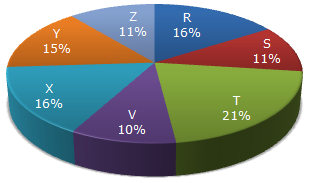# Data Interpretation - Pie Charts - Discussion

Study the following pie-chart and the table and answer the questions based on them.

Proportion of Population of Seven Villages in 1997Village % Population Below Poverty Line X 38 Y 52 Z 42 R 51 S 49 T 46 V 58

4.

If in 1998, the population of villages Y and V increase by 10% each and the percentage of population below poverty line remains unchanged for all the villages, then find the population of village V below poverty line in 1998, given that the population of village Y in 1997 was 30000.

 [A]. 11250 [B]. 12760 [C]. 13140 [D]. 13780

Explanation:

Population of village Y in 1997 = 30000 (given).

Let the population of village V in 1997 be v.

 Then, 15 : 10 = 30000 : vv = 30000 x 10 = 20000. 15

Now, population of village V in 1998 = 20000 + (10% of 20000) = 22000.Population of village V below poverty line in 1998 = 58% of 22000 = 12760.

 Jigme said: (Aug 21, 2010) Here it is not mentioned that the population figure of 30000 is for the village Y. while solving this problem if we take 30000 as the total population we get 1914 as the answer.

 Shambhu Nath Dey said: (Oct 13, 2010) Here mention population figure V is 30000. So V population below proprty level 19140.

 Rahul Shrivas said: (Mar 12, 2017) Is there any shortcut tricks to solve this problem.